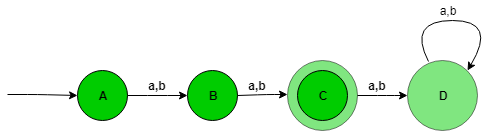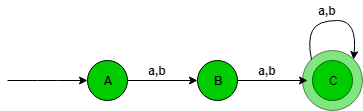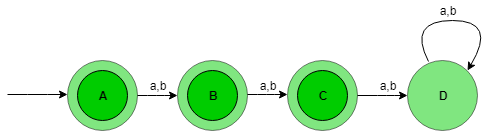TOC | Designing Deterministic Finite Automata (Set 1)

Prerequisite – Designing finite automata
In this article, we will see some designing of Deterministic Finite Automata (DFA).

Problem-1: Construction of a DFA for the set of string over {a, b} such that length of the string |w|=2 i.e, length of the string is exactly 2.

Explanation – The desired language will be like:

L = {aa, ab, ba, bb}

The state transition diagram of the language will be like:Here,
State A represent set of all string of length zero (0), state B represent set of all string of length one (1), state C represent set of all string of length two (2). State C is the final state and D is the dead state it is so because after getting any alphabet as input it will not go into final state ever.

Number of states: n+2
Where n is |w|=n

The above automata will accept all the strings having the length of the string exactly 2. When the length of the string is 1, then it will go from state A to B. When the length of the string is 2, then it will go from state B to C and when the length of the string is greater than 2, then it will go from state C to D (Dead state) and after it from state D TO D itself.

Problem-2: Construction of a DFA for the set of string over {a, b} such that length of the string |w|>=2 i.e, length of the string should be at least 2.

Explanation – The desired language will be like:

L = {aa, ab, ba, bb, aaa, aab, aba, abb........}

The state transition diagram of the language will be like:Here,
State A represent set of all sting of length zero (0), state B represent set of all sting of length one (1), and state C represent set of all sting of length two (2).

Number of states: n+1
Where n is |w|>=n

The above automata will accept all the strings having the length of the string at least 2. When the length of the string is 1, then it will go from state A to B. When the length of the string is 2, then it will go from state B to C and lastly when the length of the string is greater than 2, then it will go from state B to B itself.

Problem-3: Construction of a DFA for the set of string over {a, b} such that length of the string |w|<=2 i.e, length of the string is atmost 2. Explanation – The desired language will be like:

L = {ε, aa, ab, ba, bb}

The state transition diagram of the language will be like:Here,
State A represent set of all sting of length zero (0), state B represent set of all sting of length one (1), state C represent set of all sting of length two (2), state A, B, C is the final state and D is the dead state it is so because after getting any alphabet as input it will not go into final state ever.

Number of states: n+2
Where n is |w|<=n

The above automata will accept all the strings having the length of the string at most 2. When the length of the string is 1, then it will go from state A to B. When the length of the string is 2, then it will go from state B to C and lastly when the length of the string is greater than 2, then it will go from state C to D (Dead state).My Personal Notes arrow_drop_up